Projectile motion has been exploited many times over the centuries in many different ways, whether it was firing a cannonball on the battlefield or launching men to meander across the Moon.

Projectile motion, where an object is fired, shot, thrown or kicked up in the air, only to come down under the influence of gravity, is also important in a wide variety of sports, including many Olympic events. High Jump, Triple Jump, Pole Vault, Shot put, Javelin, Discus, Hammer, Archery, Shooting, Badminton, Showjumping, Table Tennis, Football, Beach Volleyball, Handball, Basketball and Volleyball all involve some kind of projectile motion.

Long jump also involves projectile motion and mathematics can be used to see what the maximum long jump record could be and why modern athletes can’t quite get there.

Let’s set up the problem with a diagram: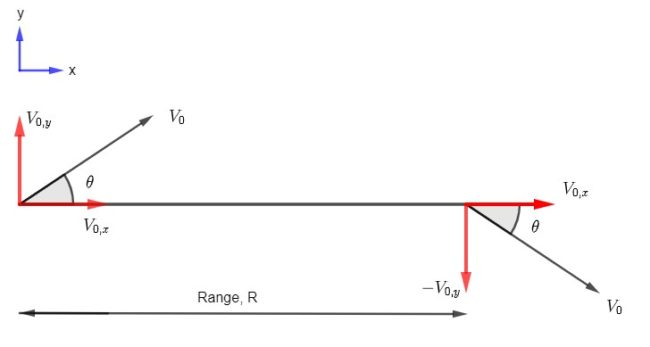A projectile (the jumper) is launched into the air at an angle, θ, to the ground with a velocity, V0. Their motion through the air is described by a parabola that is symmetric about its highest point. Once the jumper reaches this highest point they have zero velocity in the y-direction. Gravity then accelerates their motion back to the ground where they land a distance, R, from their take off point. Due to the symmetry, the velocity with which they land has the same magnitude, but opposite direction, to their take off velocity.

It is possible to derive an equation for R only in terms of the take off speed and angle.

Start by taking components of V0:

At launch:At landing:Equally re-written as: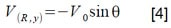and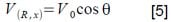Equations  and  are identical – there is no change in the x velocity, there is only an acceleration in the y-direction (due to gravity). As the velocity in the x-direction is constant throughout the flight of the projectile, we can write: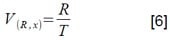where T is the duration of flight.

Substituting in from equation  gives: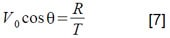Re-arranging for T gives:It is also known, from the equations of kinematics, that, for constant acceleration:where v = landing velocity, u is take off velocity and a is acceleration.

Substituting in the y-components of velocity from  and  gives: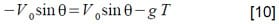Where a has been replaced with –g the acceleration due to gravity.

Further substituting in for T from equation  gives:We are after the range and so re-arranging the equation for R gives:To tidy this up we can use the following trigonometrical identity: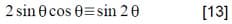Plugging into equation  yields: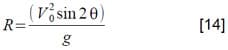This gives what is known as the ‘range equation’ for projectiles: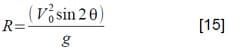Note how the maximum value of R occurs when θ = 45º (sin 90 = 1).

We can now use this equation to look at the Long Jump.

### Long Jump

During the Long Jump an athlete sprints down a track towards a marker. Before reaching the marker they have to the take off before landing in a pit of sand. The winner is the person who jumps furthest from the marker – i.e. whose range is the longest.

A top athlete can reach take off speeds of around 10 metres per second. Taking that figure, along with an optimum take off angle of 45º, and plugging them into the range equation gives:The current Men’s World Record, held by American Mike Powell, stands at 8.95 metres. The reason for this discrepancy is that it isn’t possible to take off at 45º.

A 45º take off would imply equal horizontal and vertical components of velocity (in metres per second)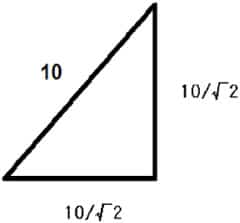A vertical component of 7.07 metres per second is not possible. A reasonable guess for the actual take off angle of a long jumper would be 25º.

Long jumpers can also sprint pretty fast – most male athletes who run in the Decathlon (a combination of ten track and field events including Long Jump and Sprinting) complete the 100 metres in around 10.3 seconds. This gives their average sprinting speed as 9.71 metres per second.

However, there is a catch: the centre of mass of the long jumper, which isn’t far from their belly button, starts approximately 1 metre above the ground. So we can’t just use the sprinting speed and angle above; we need to derive a modified version of the range equation.

When the athlete lands in the sand pit their centre of mass is lowered to ground level. This means the athlete’s centre of mass travels further on the way down than on the way up. During this extra travel time it also continues to travel horizontally.

This is easier to see by re-drawing the diagram.How far does the centre of mass actually travel horizontally, the true range, R?

By re-arranging equation , and looking horizontally only, we can say that: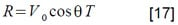So what we need to know is the total time the jumper is in the air for.

We can say that: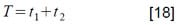where t1 is the time from ground to the highest point of the flight and t2 is the time from that highest point back to the ground.

At the highest point of the flight the jumper has zero velocity in the vertical direction. Combining that with equation  gives: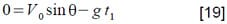Re-arranging gives: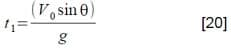The vertical distance travelled from this highest point to the ground is s+h in the diagram.

Using an equation of kinematics we can say that: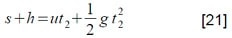where u is the initial velocity of the jumper’s centre of mass. As the initial velocity of the fall from the top of the climb is zero, this simplifies to just: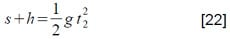Re-arranging for t2 gives: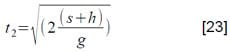However, we don’t know s and so need to write it in terms of variables we do know.

Once again looking vertically we know from the equation of kinematics that: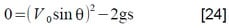Re-arranging for s gives:Plugging into equation  gives: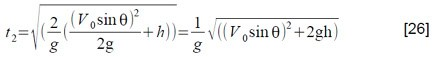Plugging equations  and  into equation  gives: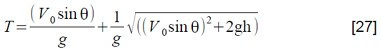Plugging that into equation  gives:Using equation  and doing some tidying finally gives: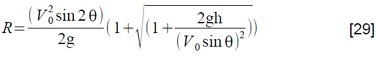We have the original range equation multiplied by a factor written only in terms of variables we know. V0 is approximately the same as the sprinting speed of the athlete: around 9.71 ms-1. The angle of take off is around 25º and the initial height of the athlete’s centre of mass is approximately 1 metre.
Plugging this values into equation  gives: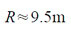This is around half metre further than Powell’s current world record. This is because, in reality, there are other factors to consider like air resistance etc. In fact, long jump world records are always quoted alongside a figure for the wind velocity at the time.

Article by Colin Stuart